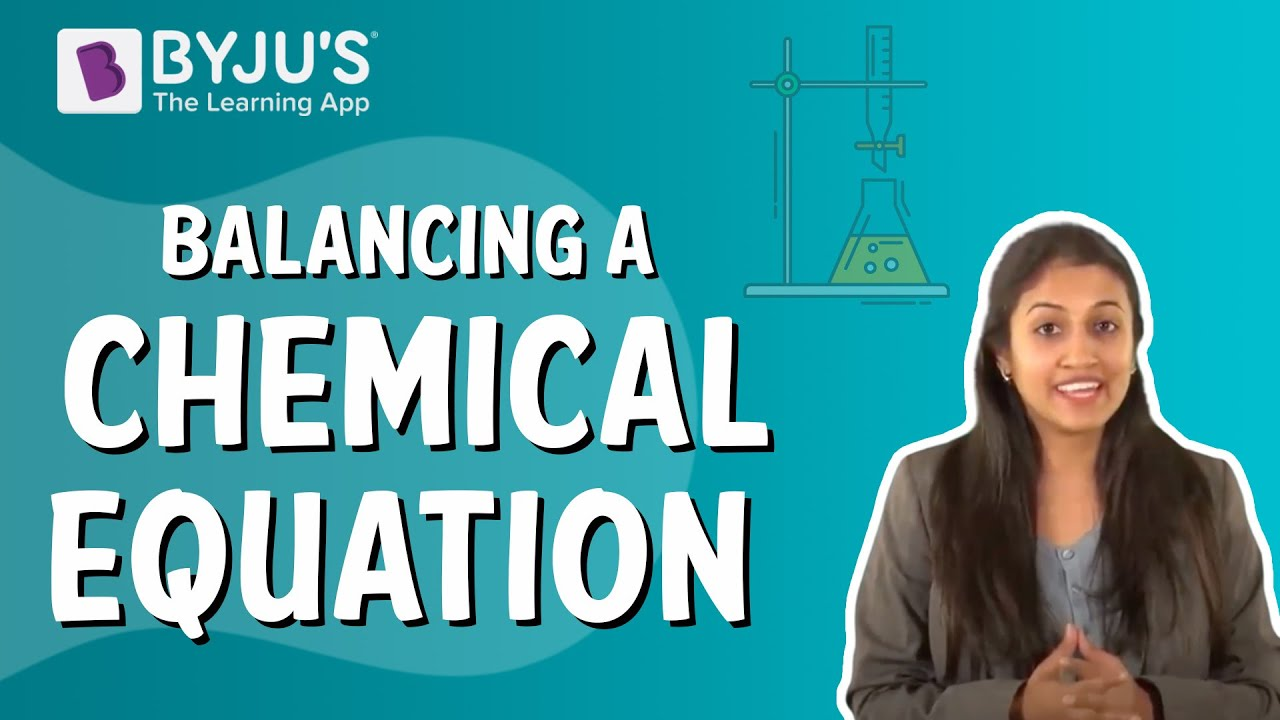# How to balance chemical equations?

Chemical equations are symbolic representations of chemical reactions in which the reactants and the products are expressed in terms of their respective chemical formulae. Chemical reactions can be represented on paper with the help of chemical equations, an example for which is represented below

Example – For the reaction between hydrogen gas and oxygen gas to form water.

2H2 + O2 → 2H2O

## Balancing chemical equation

Balancing chemical equation refers to balancing the stoichiometric coefficients on the reactants and products side. This must be done as the chemical equation obeys the law of conservation of mass and momentum.

When it comes to balancing chemical equations, the first step is to obtain the full imbalanced equation. The combustion process between propane and oxygen is used as an example to demonstrate this method.

Step 1: The unbalanced chemical equation can be written as C3H8 + O2 → CO2 + H2O

Step 2: The following table shows the number of atoms on each side.

 Reactant Side Product Side 3 Carbon atoms from C3H8 1 Carbon atom from CO2 8 Hydrogen atoms from C3H8 2 Hydrogen atoms from H2O 2 Oxygen atoms from O2 3 Oxygen atoms, 2 from CO2 and 1 from H2O

Step 3: Add Coefficients To Balance the Mass in a Chemical Equation

Note that the coefficients that are to be added must be a whole number and one coefficient added applies to all the atoms present in that one particular molecule.

 Reactant Side Product Side 3 Carbon atoms from C3H8 3 Carbon atoms from CO2 8 Hydrogen atoms from C3H8 8 Hydrogen atoms from H2O 10 Oxygen atoms from O2 10 Oxygen atoms, 6 from CO2 and 4 from H2O

∴ The balanced chemical equation is C3H8 + 5O2 → 3CO2 + 4H2O(57)(8)